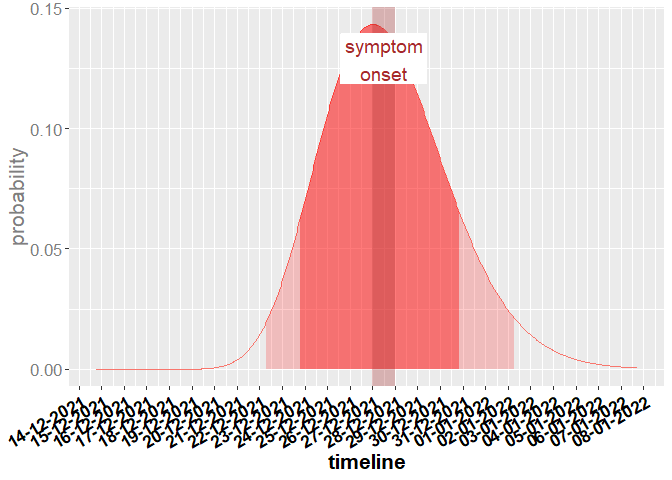# Statistical Modelling for Infectious Disease Management - Contagious period

## Question

A person begins to show symptoms of COVID-19 on 28.12.2021. When has the person been infectious?

## Generating a data frame with dates and infectiousness probability using get_infectiousness_density

If the person shows symptoms, the function get_infectiousness_density() can be used to answer the question. The function get_infectiousness_density() creates a dataframe containing the infectiousness at a particular date/time.

### Inputs

The following input arguments are needed for the function get_infectiousness_density():

The symptom_begin_date is the date when the person started to have symptoms.

Then, the max_infectious_days is needed, which defines the interval length of the distribution output.

The other three inputs infectiousness_shift, shape_infectiousness_gamma and rate_infectiousness_gamma are the parameters of the gamma distribution for the infectiousness profile.

symptom_begin_date <- as.Date("2021-12-28")
infectiousness_shift <- 12.272481
max_infectious_days <- 24
shape_infectiousness_gamma <- 20.516508
rate_infectiousness_gamma <- 1.592124

infectious_df <- get_infectiousness_density(symptom_begin_date,
infectiousness_shift,
max_infectious_days,
shape_infectiousness_gamma,
rate_infectiousness_gamma)

### Methodology

The default values of the gamma distribution are taken from the paper He et al . In this paper an analysis of COVID-19 viral shedding and transmissibility was conducted and a gamma distribution for the infectious period of cases was estimated based on their symptom onset dates.

### Output

The function call returns the following data frame:

values 100 to 109 of resulting data frame
dates distribution
100 2021-12-19 21:27:37 0.0000353
101 2021-12-19 22:27:37 0.0000402
102 2021-12-19 23:27:37 0.0000457
103 2021-12-20 00:27:37 0.0000519
104 2021-12-20 01:27:37 0.0000587
105 2021-12-20 02:27:37 0.0000663
106 2021-12-20 03:27:37 0.0000748
107 2021-12-20 04:27:37 0.0000843
108 2021-12-20 05:27:37 0.0000947
109 2021-12-20 06:27:37 0.0001063

The function get_infectiousness_density() creates an interval of length max_infectious_days and the resulting data frame shows the resulting density of the gamma distribution for each hour of the period. This density can be used for calculating the most probable period to infect other people.

## Visualization example of the data frame of get_infectiousness_density

The following code generates a plot with the gamma distribution of the infectiousness profile and the 80% and 95% high density intervals.

Source
.calculate_qstart_qend <- function(probability, df) {
hdr_df <- hdr(den = data.frame(x = 1:length(df$distribution), y = df$distribution), p = probability * 100)$hdr qstart <- (hdr_df[1, 1] - 1) / 24 qend <- (hdr_df[1, 2] - 1) / 24 return(list("qstart" = qstart, "qend" = qend)) } period_80 <- .calculate_qstart_qend(0.8, infectious_df) period_95 <- .calculate_qstart_qend(0.95, infectious_df) Source .shade_curve <- function(df, qstart, qend, fill = "red", alpha = 0.4) { subset_df <- df[floor(qstart * 24):ceiling(qend * 24), ] geom_area(data = subset_df, aes(x = x, y = y), fill = fill, color = NA, alpha = alpha) } Source  symptom_begin_date <- as.Date("2021-12-28") df <- infectious_df symp_date_posixct_start <- as.POSIXct(format(as.POSIXct(symptom_begin_date, tz = "CET"), "%Y-%m-%d")) symp_date_posixct_end <- as.POSIXct(format(as.POSIXct(symptom_begin_date + 1, tz = "CET"), "%Y-%m-%d")) symp_date_posixct_mid <- symp_date_posixct_start - as.numeric(difftime(symp_date_posixct_start, symp_date_posixct_end, units = "hours")) / 2 * 3600  Source  g <- ggplot() + scale_x_datetime(breaks = scales::date_breaks("1 days"), labels = scales::date_format("%d-%m-%Y")) + theme(axis.text.x = element_text(angle = 90)) + # scale_x_continuous(breaks = x_tick, # labels = x_label) + # theme(axis.ticks.x = element_line(color = c(rbind(rep("black", length(x_label)/2), rep(NA, length(x_label)/2))), linetype = 2, size = 1))+ geom_path(aes(x = df$dates, y = df$distribution, color = "red")) + .shade_curve(df = data.frame(x = df$dates, y = df$distribution), period_80$qstart,
period_80$qend) + .shade_curve(df = data.frame(x = df$dates, y = df$distribution), period_95$qstart,
period_95$qend, alpha = 0.2) + geom_rect(data = data.frame(xmin = symp_date_posixct_start, xmax = symp_date_posixct_end, ymin = -Inf, ymax = Inf), aes(xmin = xmin, xmax = xmax, ymin = ymin, ymax = ymax), fill = "brown", alpha = 0.3) + geom_label(aes(x = symp_date_posixct_mid, y = 0.9*max(df$distribution), label = "symptom\nonset"),
colour = "brown", fill = "white", size = 5, label.size = NA) +
ylab("probability") +
xlab("timeline") +
labs(color = 'Verteilung') +
# ggtitle("Visualization of get_infection_density") +
theme(legend.position = "none", text = element_text(size = 16*5/5)) +
theme(axis.text.x = element_text(colour = "black", face = "bold", angle = 30, hjust = 1)) +
theme(axis.title.x = element_text(colour = "black", face = "bold")) +
theme(axis.text.y = element_text(colour = "gray50")) +
theme(axis.title.y = element_text(colour = "gray50"))

g## Literature

 He X, Lau EHY, Wu P, Deng X, Wang J, Hao X, Lao YC, Wong JY, Guan Y, Tan X, Mo X, Chen Y, Liao B, Chen W, Hu F, Zhang Q, Zhong M, Wi Y, Zhao L, Zhang F, Cowling BJ, Li F, Leung GM. Temporal dynamics in viral shedding and transmissibility of COVID-19. Nature Medicine. 2020; 26: 672–675.